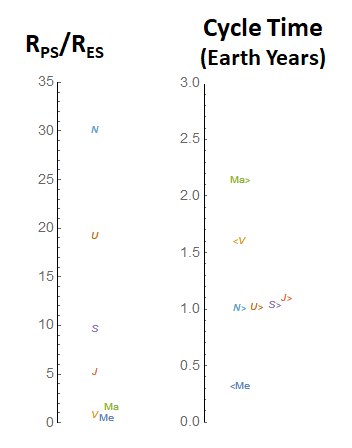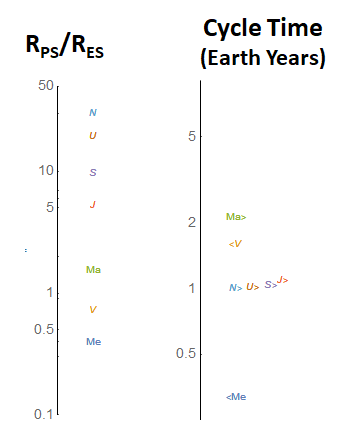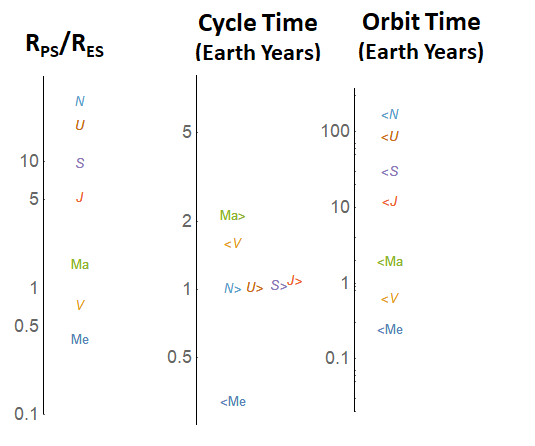# How You Can Determine Each Planet’s Year from its Distances and Cycles

Whether you’re a die-hard who insists on measuring the distances between the planets and the Sun yourself (which I’ve shown you how to do here and here), or you are willing to accept what other people tell you about them, it’s interesting to look at the pattern among these distances. They are shown at right, starting with the smallest — Mercury (Me) — and proceeding to Venus (V), Mars (Ma), Jupiter (J), Saturn (S), Uranus (U) and Neptune (N), nearly 100 times further out than Mercury. The inner planets up to Mars are very close together, all bunched within 1.5 times the Earth-Sun distance, whereas the outer planets are much further apart.Figure 1: (Left) the planet-Sun distances relative to the Earth-Sun distance; (Right) the time between solar conjunctions, when the planet disappears (roughly) behind the Sun from Earth’s perspective; “>” indicates the planet moves into the morning sky (east) after conjunction, whereas “<” means it moves into the evening sky (west).

Also shown in the figure are the lengths of the planet’s cycles. Remember, a cycle starts when a planet reappears from behind the Sun and ends when a planet again disappears behind the Sun… the moment of “solar conjunction,” or just “conjunction” for short in this post. Some planets have short cycles, others have long ones. Interestingly, now it is the outer planets that all bunch up together, with their cycles just a bit longer than an Earth year, whereas Mercury, Venus and Mars have wildly different cycles ranging from a third of an Earth-year to two Earth-years. In the figure I’m also keeping track of something that I didn’t mention before. As their cycles begin, Mercury and Venus initially move into the evening sky, in the west, setting just after sunset. I’ve indicated that with a “<” Meanwhile Mars, Jupiter and Saturn move into the eastern morning sky, rising just before sunrise, as indicated with a “>”. (Mars, Jupiter and Saturn just reappeared from behind the Sun this winter; that’s why they’re all in the morning sky right now.) This difference is going to prove important in a moment.

Before going on, let me make another version of the same figure, easier to read. This involves making a “logarithmic plot”. Instead of showing the step from 1 to 2 as the same as the step from 0 to 1, as we usually do, we replot the information so that the step along the axis from 1 to 10 is the same as the step from 0.1 to 1. It’s gives exactly the same information as the Figure 1, but now the planet-Sun distances don’t bunch up as much.

### Orbits Vs. CyclesFigure 2: exactly the same as Figure 1, but with the vertical axis in “log-plot” form.

Now, the cycles from one solar conjunction to the next, long beloved of astrologers, are not beloved of astronomers, because they involve a combination of two physically unrelated motions. A solar conjunction happens when a planet disappears behind the Sun from Earth’s perspective, so the time between one conjunction and the next combines:

• the orbital motion of the planet around the Sun;
• the yearly rotation of the line between the Sun and the Earth. (So far, we haven’t found evidence as to whether the Sun moves around the Earth or the Earth moves around the Sun — and we’ll remain agnostic about that today.)

So what astronomers want to know is the orbital period of each planet — it’s own year. That is, how long does it takes each planet to orbit the Sun, from the planet’s perspective, or from the Sun’s perspective. This is the time that an observer on the Sun would see for the planet to complete a circle relative to the fixed stars, and vice versa. (Remember we gathered evidence that the stars are fixed, or extremely slowly drifting from the perspective of the Earth, using a gyroscope, whereas either or both the Sun or the Earth are rotating relative to one another by about one degree per day. We also know the stars are much further than the Sun from our two measurements of the Moon’s radius.)

### The Orbits: Some Qualitative Facts

Knowing the time of a planet’s cycle in Earth-years, how can we figure out its orbital period? This takes a little thinking and a tiny bit of algebra.

For any planet, there are three possibilities to think about. Relative to the rotating Earth-Sun line, the planet might

• orbit the Sun in the opposite direction
• orbit the Sun in the same direction but slower than the Earth-Sun line rotates
• orbit the Sun in the same direction but faster than the Earth-Sun line rotates

Let’s first understand what would happen in the seemingly absurd but useful case where the planet does not move at all. Then the planet’s cycle would be exactly one Earth-year, because after each conjunction, the next would occur after the Earth-Sun line rotated exactly once. Less obviously, the planet would appear, right after a conjunction, in the eastern sky, just before sunrise; that will be visible in the next figure.

If a planet moved opposite to the Earth-Sun line — so that, as I have drawn it in Figure 3, the planet moves clockwise while the Earth-Sun line moves counter-clockwise — then the time between its conjunctions must be less than one Earth-year. That’s because planet will again disappear behind the Sun before it does a full circle; an example is shown in the picture. But just as in the stationary case, it moves (from the Earth’s perspective) into the eastern sky right after conjunction. If you refer to Figure 2, you’ll see that Mercury’s the only planet with a cycle less than one Earth-year, but it appears in the western sky right after conjunction. Therefore, no planet moves opposite to the Earth-Sun line: all of the planets orbit the Sun in the same direction.Figure 3: A planet orbiting in the opposite direction as the Earth-Sun line rotates would appear in the eastern sky after solar conjunction (left) and have a second conjunction in less than an Earth-year (right). As can be seen from Figure 2, there is no such planet.

What if the planet orbits in the same direction as the Earth-Sun line’s rotation, but more slowly? Then, because the planet can’t keep up with the Earth-Sun line, it will again appear in the eastern sky right after conjunction. That rules out Venus and Mercury. And how long is its cycle? After one Earth-year, the Earth-Sun line comes back to its starting point, but the planet will have moved along in its orbit by then, so the Earth-Sun line has to rotate further before the planet can pass behind the Sun. Therefore its cycle will take more than one Earth-year. Fitting this description — see Figure 2 — are Mars, Jupiter, Saturn, Uranus and Neptune.Figure 4: A planet orbiting in the same direction as the Earth-Sun line’s rotation, but more slowly, would appear in the eastern sky after solar conjunction (left) and have a second conjunction in more than an Earth-year (right), when the Earth-Sun line has completed one more orbit than the planet. As can be seen from Figure 2, this is true of Mars through Neptune.

By reversing the logic, we can see that if the the planet orbits in the same direction as, but faster than, the Earth-Sun line’s rotation, then it must appear in the western sky immediately following conjunction. Well, Mercury and Venus fit that bill.Figure 5: A planet orbiting in the same direction as the Earth-Sun line’s rotation, but more slowly, would appear in the western sky after solar conjunction (left). As can be seen from Figure 2, this is true of Mercury and Venus. Its second conjunction occurs after the planet has made one more orbit than the Earth-Sun line has.

That tells us the status of all of the sky’s planets:

• the near Sun-orbiting planets orbit more quickly than the Earth-Sun line rotates, and so they have an orbit (though not necessarily a cycle!) that lasts less than one Earth-year,
• the far Sun-orbiting planets orbit more slowly than the Earth-Sun line rotates, and so they have an orbit that last more than one Earth-year.

That’s already a nicer pattern than the cycles display!

### The Orbits: Quantitative Details

Knowing this, let’s first determine the orbital period for Mars and the far Sun-orbiters. We saw in Figure 4 that if we refer to a planet’s cycle as lasting C Earth-years, then for these slowly orbiting planets, C>1. The Earth-Sun line rotates by 360 degrees per Earth-year, and during the cycle it will have rotated by 360*C degrees. That’s more than a full circle. What about the planet? It’s been trying but failing to keep up with the Earth-Sun line, so it has orbited by a smaller angle. Conjunction happens when it has fallen behind by exactly 360 degrees! That means that during one cycle, while the Earth-Sun line has rotated by 360*C degrees, the planet has moved around its orbit by 360*C – 360 = 360*(C-1) degrees. See Figure 6 below.

How long does it take the planet completes a full circle around the Sun? We just saw that it takes the planet C Earth-years to move 360*(C-1) degrees:

• 360*(C-1) degrees = C Earth-years

But a full orbit of the Sun — a full circle — requires the planet to move by 360 degrees. So divide both sides by (C-1) to get

• 360 degrees = C/(C-1) Earth-years per orbit

That’s the formula we need for the planets from Mars out to Neptune.Figure 6: A slower planet reaches a second conjunction when it has traveled one fewer orbit than the Earth-Sun line (left); a faster planet does so after traveling one additional orbit (right).

For the near Sun-orbiters Mercury and Venus, which move faster than the Earth, the logic is simply reversed. They run ahead of the Earth-Sun line, and so, after one conjunction, they won’t have a second one until they’ve outrun the Earth-Sun line by 360 degrees. So if such a planet’s cycle takes C Earth-years, during which the Earth-Sun line has rotated by 360*C degrees, the planet must have moved by an extra 360 degrees, and thus a total of 360*C+360 = 360*(C+1) degrees. The same logic then implies that if

• 360*(C+1) degrees = C Earth-years

for this planet, a full orbit requires

• 360 degrees = C/(C+1) Earth-years per orbit.

That’s the formula we need for the two innermost planets.

### The Orbits: A Discovery!

Okay! So now it’s just a matter of applying these formulas to the cycle times: if the cycle takes C Earth-years, then the orbit time is

• T = C/(C+1) Earth-years for Mercury and Venus;
• T = C/(C-1) Earth-years for the other planets.

And when we use those formulas, we find:

where for Cycles “E” means the planet moves to the east of the Sun from Earth’s perspective after a conjunction, and “W” that it moves to the west, while for Orbits “W” means all planets orbit in the same direction as the Earth-Sun line rotates.

(If you’d really done this yourself, the numbers you’d obtain wouldn’t likely be as close as these values are to the true ones, especially for Uranus and Neptune and even Saturn. You would likely be off by quite a bit! But your results would be pretty close for the inner planets, and qualitatively right even for the far outer planets. If you wanted to do better, you’d need more precise measurements.)

Now let’s remake Figure 2 but now showing the planet-Sun distances, the cycle times, and the orbital times. Whoa! Something very interesting has happened!Figure 7: same as Figure 2, with the addition of the planets’ orbital times in Earth years, as calculated from the cycles using the formulas above. All the planets orbit in the same direction “<“, and there is a similar pattern for their orbital periods and their distances from the Sun.

The plots of the distances and the orbital times, unlike that of the cycle times, look remarkably similar! Not only is there a general trend, where the planets further out take longer to orbit, they are spaced in the same way both in their distances to the Sun and in their orbital periods.

What does this similarity mean? It can’t be an accident! Next time we’ll figure that out, and consider the implications.

### 2 thoughts on “How You Can Determine Each Planet’s Year from its Distances and Cycles”

1.Since those are log plots, it sure looks like if the scales were in ratio 3:2
they would line up perfectly? Why not draw them that way?

•Why, indeed, did it take Kepler many years to do that? And why didn’t Copernicus do it decades earlier, since he had data no less accurate than what we’ve obtained here, out to Saturn?